# Relationship of Frequency Vs KVA Output or Rating of Transformer

### Relationship of Frequency Vs KVA output or Rating of the transformer.

When we purchase a transformer it is always marked in that name plate is that is designed to operate for particular frequency. Even though in transformer, the frequency is unchanged, but why we have to care about input frequency of the transformer. Let see the relationship between the transformer and KVA output of the transformer,

As you know the AC(Alternating current) input voltage of the transformer V1 & the output secondary voltage of the transformer V2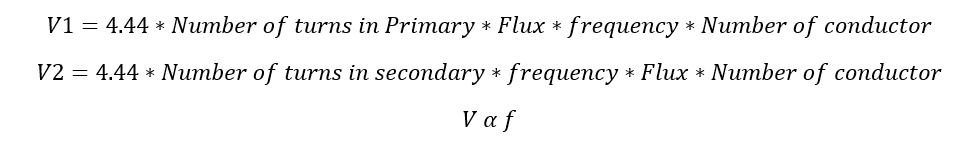Here the flux, frequency & number of conductor are constant

The transformer output Q1 is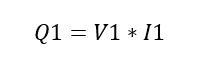I1 is the flow of current in the primary winding.

Always transformers are rating KVA. See Why.

Apply the voltage equation in the output equation of the transformer.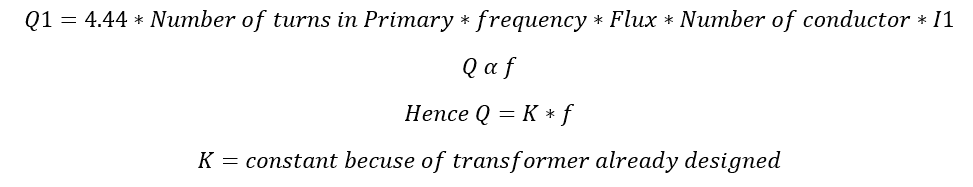Also from the transformer KVA design data.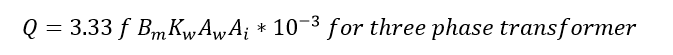Here you can see the KVA output of the transformer is directly proportional to the input frequency of the transformer. When you decrease the frequency the KVA output considerably decreases and if you increase the frequency then the KVA output of the transformer will get increase. At that same time the eddy current losses and hysteresis losses increases as that of increasing frequency of the transformer. But another one important fact that while changing the frequency of the transformer you should change conductor size to carry the KVA output without increasing the voltage. If you are increasing the voltage, then you do not need to increase size of the conductor.

But, to operate the transformer in same voltage, same size of the conductor and different frequency we should reduce the KVA output of the same transformer.

### Frequency Vs kVA Rating of the transformer

Let see a Simple example.

Now a three phase, 500 KVA, 60 Hz transformer is operating at the voltage of 11000 V/440 V. Now we are planning to operate the same transformer at 50 Hz and find out the reduction KVA.

Consider

Q1 = existing KVA for 60 Hz operating

F1= frequency of existing transformer.

Q2 = Modified transformer KVA output

F2= changing the frequency.

K = constant because the transformer already designed.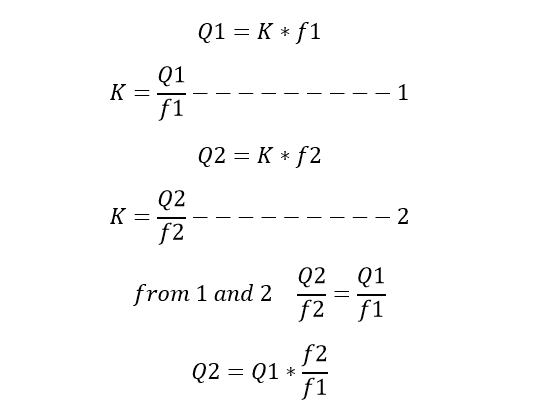Apply the transformer data. On the equation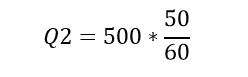After solving the equation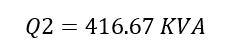The KVA considerably reduced when you decreasing the transformer input frequency.

Note: This is the condition, that you should operate a transformer with the same design, without changing the insulation or conductor size.

Now you will get an another question, by increasing the frequency of the same transformer i.e 60 Hz to 70 Hz does it increase the KVA output ?… Yes of course. It increases the KVA output. At the same time, the core losses also increases. Eddy current loss is directly proportional to the frequency and hysteresis losses is directly proportional to the square of the frequency. To tackle this losses, you should decrease the input frequency of the transformer or you should make necessary cooling arrangement to get stable operation.

Learn More:   Line Voltage to Phase Voltage Line Current to Phase Current Relationship